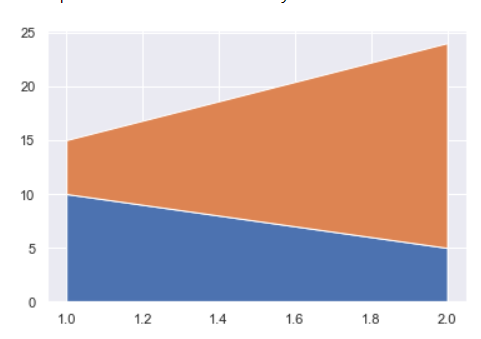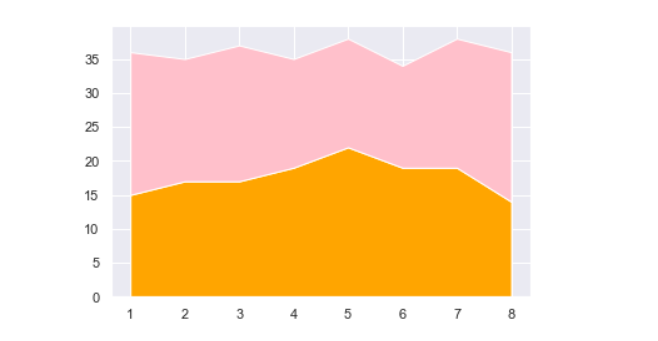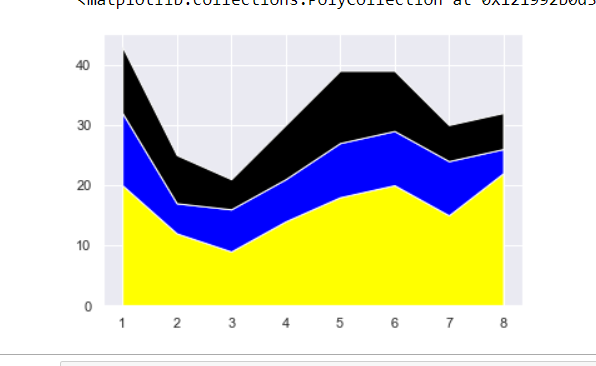# How to Create an Area Chart in Seaborn?

• Last Updated : 19 Dec, 2021

In this article, we are going to see how to create an area chart in seaborn using Python.

Area Charts are a great way to quickly and easily visualize things to show the average overtime on an area chart. Area charts are different from line graphs. Area charts are primarily used for the summation of quantitative data. however, the area between the line and the area chart is filled in with shading or color.

Area graph displays graphically quantitative data. It is based on the line chart. In both line graphs and area charts, data points are connected by line segment to show the values of a quantity at different points.

### Example 1: Create Basic Area Chart in Seaborn

In this example, we are going to see how to create a basic area chart in seaborn.

## Python3

 `import` `pandas as pd ` `import` `matplotlib.pyplot as plt ` `import` `seaborn as sns ` ` `  `#set seaborn style ` `sns.set_theme() ` ` `  `#define DataFrame ` `data ``=` `{``'period'``: [``1``, ``2``], ` `                   ``'rohit'``: [``10``, ``5``], ` `                   ``'vikey'``: [``5``, ``19``], ` `                   ``} ` ` `  `df ``=` `pd.DataFrame(data) ` ` `  `#create area chart ` `plt.stackplot(df.period, df.rohit, df.vikey, ` `              ``labels``=``[``'rohit'``, ``'vikey'``])`

Output:### Example 2: Create Custom Area Chart in Seaborn

In this example, we are going to see how to create an area chart by defining different colors in color_map=[] in seaborn.

## Python3

 `import` `pandas as pd ` `import` `matplotlib.pyplot as plt ` `import` `seaborn as sns ` ` `  `# set seaborn style ` `sns.set_theme() ` ` `  `data ``=` `{``'period'``: [``1``, ``2``, ``3``, ``4``, ``5``, ``6``, ``7``, ``8``], ` `                   ``'team_B'``: [``15``, ``17``, ``17``, ``19``, ``22``, ``19``, ``19``, ``14``], ` `                   ``'team_C'``: [``21``, ``18``, ``20``, ``16``, ``16``, ``15``, ``19``, ``22``]} ` ` `  `# define DataFrame ` `df ``=` `pd.DataFrame(data) ` ` `  `# define colors to use in chart ` `color_map ``=` `[``'orange'``, ``'pink'``] ` ` `  `#create area chart ` `plt.stackplot(df.period, df.team_B, df.team_C, ` `              ``labels``=``[``'Team B'``, ``'Team C'``], ` `              ``colors``=``color_map)`

Output:### Example 3: Create Multiple Area Chart in Seaborn.

In this example, we are going to see how we can add create multiple area charts in a single subplot by defining different data with its different color in color_map=[] in seaborn.

## Python3

 `import` `pandas as pd ` `import` `matplotlib.pyplot as plt ` `import` `seaborn as sns ` ` `  `# set seaborn style ` `sns.set_theme() ` `  `  `# define DataFrame ` `df ``=` `pd.DataFrame({``'Class'``: [``1``, ``2``, ``3``, ``4``, ``5``, ``6``, ``7``, ``8``], ` `                   ``'sec_A'``: [``20``, ``12``, ``9``, ``14``, ``18``, ``20``, ``15``, ``22``], ` `                   ``'sec_B'``: [ ``12``, ``5``, ``7``, ``7``, ``9``, ``9``, ``9``, ``4``], ` `                   ``'sec_C'``: [``11``, ``8``, ``5``, ``9``, ``12``, ``10``, ``6``, ``6``]}) ` ` `  `# define colors to use in chart ` `color_map ``=` `[``'yellow'``, ``'blue'``, ``'black'``] ` ` `  `# create area chart ` `plt.stackplot(df.Class, df.sec_A, df.sec_B, df.sec_C, ` `              ``labels``=``[``'Sec A'``, ``'Sec B'``, ``'Sec C'``], ` `              ``colors``=``color_map)`

Output:My Personal Notes arrow_drop_up
Recommended Articles
Page :